# Averaging

(diff) ← Older revision | Latest revision (diff) | Newer revision → (diff)

The operation of calculating the mean values of functions forming part of the structure of differential equations which describe periodic, almost-periodic, and, generally, oscillating processes. The operation of averaging can be considered as a type of smoothing operator. Averaging methods first came into use in celestial mechanics in the study of planetary motion around the Sun. Later, they propagated to a wide variety of areas: to the theory of non-linear oscillations, to physics, to the theory of automatic control, to astrodynamics, and to others. Averaging methods have often provided approximate solutions for the initial equations. The most typical classes of differential equations for which averaging methods are used are the following.

1) Standard systems in the sense of N.N. Bogolyubov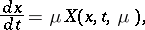(1)

whereare vectors,is the time, andis a small positive parameter.

2) Multi-frequency autonomous-periodic systems(2)

whereare vectors,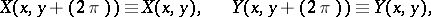and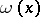is the frequency vector.

3) Multi-frequency non-autonomous systems(3)

Instead of the systems (1)–(3), "simpler" averaged systems of a first approximation are considered: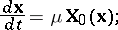(1prm)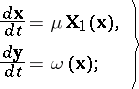(2prm)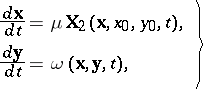(3prm)

where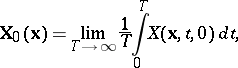(4)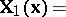(5)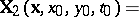(6)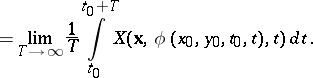The formulas (4)–(6) express the most widespread averaging methods.

Formula (6) expresses the scheme of averaging "along the generating solution" . In the function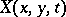, the vectoris initially substituted by the generating solution of the system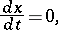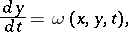after which the integral average (6) is calculated.

The principal question which arises when the systems (1)–(3) are changed is the construction of-estimates for the normson the largest possible interval (of order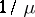) of time, if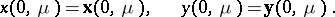This is the essence of the problem of averaging methods. For systems (1), this problem of averaging methods was proposed by Bogolyubov, whose results formed the basis of the modern algorithmic theory of ordinary differential equations.

How to Cite This Entry:
Averaging. Encyclopedia of Mathematics. URL: http://encyclopediaofmath.org/index.php?title=Averaging&oldid=12772
This article was adapted from an original article by E.A. Grebenikov (originator), which appeared in Encyclopedia of Mathematics - ISBN 1402006098. See original article